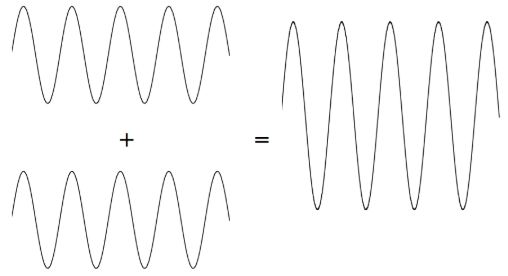$$\newcommand{\vecs}{\overset { \rightharpoonup} {\mathbf{#1}} }$$ $$\newcommand{\vecd}{\overset{-\!-\!\rightharpoonup}{\vphantom{a}\smash {#1}}}$$$$\newcommand{\id}{\mathrm{id}}$$ $$\newcommand{\Span}{\mathrm{span}}$$ $$\newcommand{\kernel}{\mathrm{null}\,}$$ $$\newcommand{\range}{\mathrm{range}\,}$$ $$\newcommand{\RealPart}{\mathrm{Re}}$$ $$\newcommand{\ImaginaryPart}{\mathrm{Im}}$$ $$\newcommand{\Argument}{\mathrm{Arg}}$$ $$\newcommand{\norm}{\| #1 \|}$$ $$\newcommand{\inner}{\langle #1, #2 \rangle}$$ $$\newcommand{\Span}{\mathrm{span}}$$ $$\newcommand{\id}{\mathrm{id}}$$ $$\newcommand{\Span}{\mathrm{span}}$$ $$\newcommand{\kernel}{\mathrm{null}\,}$$ $$\newcommand{\range}{\mathrm{range}\,}$$ $$\newcommand{\RealPart}{\mathrm{Re}}$$ $$\newcommand{\ImaginaryPart}{\mathrm{Im}}$$ $$\newcommand{\Argument}{\mathrm{Arg}}$$ $$\newcommand{\norm}{\| #1 \|}$$ $$\newcommand{\inner}{\langle #1, #2 \rangle}$$ $$\newcommand{\Span}{\mathrm{span}}$$$$\newcommand{\AA}{\unicode[.8,0]{x212B}}$$

When two waves of the same type come together it is usually the case that their amplitudes add. So two overlapping water waves have an amplitude that is twice as high as the amplitude of the individual waves. In the picture below the waves arrive in phase or with a phase difference of zero (the peaks arrive at the same time). This is called constructive interference.Figure $$\PageIndex{1}$$

In the picture below the waves are exactly 180 degrees out of phase so that the peak of one wave lies in the trough of a second wave. This is called destructive interference and the waves cancel. Noise canceling technology in cars and stereo headphones use this idea. Incoming noise is sampled and some electronics creates an identical sound wave except with a phase difference of 180 degrees so the two waves cancel. Destructive interference can also occur for stereo speakers if they are not wired properly. If the wires to one speaker are accidentally twisted the sounds from the speakers will be out of phase and tend to sound softer (the destructive interference isn't complete because of echoes and different distances to the listener).Figure $$\PageIndex{2}$$

Two other places where two waves combine to give interesting effects are standing waves and beats. When a guitar string is plucked two waves move out from the contact point in opposite directions. These waves hit the ends where the strings are tied and reflect. The result is a standing wave where the motion is up and down (you no longer see a wave moving to the left or right on the string). As we will see, standing waves determine the fundamental frequency of stringed instruments.

If two waves don't have exactly the same wavelength they may start out in phase (constructive) but gradually end up out of phase (destructive). This repeats over time and is known as the phenomena of beats. Two sound waves with slightly different frequencies will have slightly different wavelengths (remember frequency times wavelength equals speed which is fixed so sound waves of different frequencies also have different wavelengths but travel at the same speed). So at times they will reinforce each other and at other times tend to cancel. What you will hear is a single note that gets louder and softer. The beat frequency (the frequency of the loudness and softness) is the difference between the two original frequencies. So a $$500\text{ Hz}$$ sound together with a $$502\text{ Hz}$$ sound will sound like a single note of $$501\text{ Hz}$$ but with a varying loudness that changes every $$2\text{ Hz}$$. Standing waves and beats are best demonstrated in videos and simulations, below.

## Video/audio examples:

This page titled 6.6.1: Adding Waves is shared under a CC BY-NC-SA 3.0 license and was authored, remixed, and/or curated by Kyle Forinash and Wolfgang Christian via source content that was edited to the style and standards of the LibreTexts platform; a detailed edit history is available upon request.